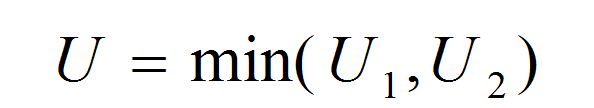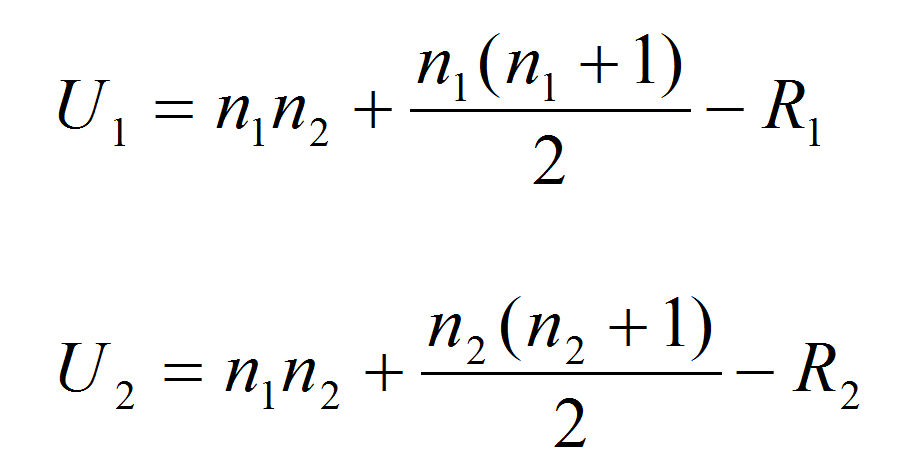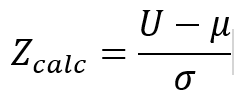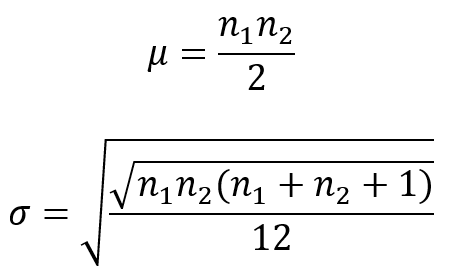# Mann Whitney Testing with JMP

### What is the Mann Whitney Test?

The Mann Whitney test (also called Mann–Whitney U test or Wilcoxon rank-sum test) is a statistical hypothesis test to compare the medians of two populations that are not normally distributed. In a non-normal distribution, the median is the better representation of the center of the distribution.

• Null Hypothesis (H0): η1 = η2
• Alternative Hypothesis (Ha): η1η2

Where:

• η1 is the median of one population
• η2 is the median of the other population
• The null hypothesis is that the medians are equal, and the alternative is that they are not equal

### Mann Whitney Test Assumptions

• The sample data drawn from the populations of interest are unbiased and representative
• The data of both populations are continuous or ordinal when the spacing between adjacent values is not constant (Reminder: Ordinal data—A set of data is said to be ordinal if the values can be ranked or have a rating scale attached. You can count and order, but not measure, ordinal data)
• The two populations are independent to each other
• The Mann–Whitney test is robust for the non-normally distributed population
• The Mann–Whitney test can be used when shapes of the two populations’ distributions are different

### How Mann Whitney Test Works

Step 1:
Group the two samples from two populations (sample 1 is from population 1 and sample 2 is from population 2) into a single data set and then sort the data in ascending order ranked from 1 to n, where n is the total number of observations.
Step 2:
Add up the ranks for all the observations from sample 1 and call it R1. Add up the ranks for all the observations from sample 2 and call it R2 .
Step 3:
Calculate the test statisticsWhere:and where:

• η1 and η2 are the sample sizes
• R1 and R2 are the sum of ranks for observations from sample 1 and 2 respectively

Step 4:
Make a decision on whether to reject the null hypothesis

• Null Hypothesis (H0): η1 = η2
• Alternative Hypothesis (Ha): η1η2

If both of the sample sizes are smaller than 10, the distribution of U under the null hypothesis is tabulated.

• The test statistic is U and, by using the Mann–Whitney table, we would find the p-value
• If the p-value is smaller than alpha level (0.05), we reject the null hypothesis
• If the p-value is greater than alpha level (0.05), we fail to reject the null hypothesis
• If both sample sizes are greater than 10, the distribution of U can be approximated by a normal distribution. In other words, (U-μ)/σ follows a standard normal distributionWhere:If the sample sizes are greater than 10, then the distribution of U can be approximated by a normal distribution. The U value is then plugged into the formula seen here to calculate a Z statistic.
When |Zcalc| is greater than Z value at α/2 level (e.g. when α = 5%, the z value we compare |Zcalc| to is 1.96), we reject the null hypothesis.

### Use JMP to Run a Mann–Whitney Test

Case study: We are interested in comparing customer satisfaction between two types of customers using a nonparametric (i.e. distribution-free) hypothesis test: Mann–Whitney test.
Data File: “Mann–Whitney.jmp”Fig 1.0 Mann-Whitney Test

• Null Hypothesis (H0): η1 = η2
• Alternative Hypothesis (Ha): η1η2

#### Steps to run a Mann–Whitney Test in JMP:

1. Click Analyze -> Fit Y by X
2. Select “Overall Satisfaction” as “Y, Response”
3. Select “Customer Type” as “X, Factor”4. Click “OK”
5. Click on the red triangle button next to “One-Way Analysis of Overall Satisfaction by Customer Type”
6. Click Nonparametric -> Wilcoxon TestModel summary: The p-value of the test is lower than alpha level (0.05); so we reject the null hypothesis and conclude that there is a statistically significant difference between the overall satisfaction medians of the two customer types.

The result of the test is boxed in: The p-value is lower than the alpha value of 0.05; therefore, we must reject the null hypothesis and claim that there is a significant difference between the median customer satisfaction levels of the two groups.